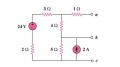# How do I Find Vth With the node voltage method?

#### student441

Joined Nov 6, 2021
11
Hello.
I'am practicing circuit analisis about the norton and thevenin's circuits and I can't seem to manage to get the right thevenin's voltage using the node voltage for a)
For the circuit in Fig. 4.111, obtain the Thevenin equivalent as seen from terminals (a) a-b (b) b-cresolution
1) I did source transformation turning the current source(2A) into a voltage source (10V)
applied node voltage discarding the 1ohm resistance.
I got that Va=18V AND VB=10V
and since vth is in parallel with the 4 ohm resistance then: Vth=(Va-Vb)/4=2 but the solution says that Vth=4
Can you help me get to the desired result.
Your time and attention are deeply appreciated.
Thank you.

#### Ramussons

Joined May 3, 2013
1,354
Are you sure that the source transformation in step 1 is correct? Does all that 2 Amps flow through the 5 Ohms resistor?

#### MrAl

Joined Jun 17, 2014
10,595
Hello.
I'am practicing circuit analisis about the norton and thevenin's circuits and I can't seem to manage to get the right thevenin's voltage using the node voltage for a)
For the circuit in Fig. 4.111, obtain the Thevenin equivalent as seen from terminals (a) a-b (b) b-c

View attachment 265342
resolution
1) I did source transformation turning the current source(2A) into a voltage source (10V)
applied node voltage discarding the 1ohm resistance.
I got that Va=18V AND VB=10V
and since vth is in parallel with the 4 ohm resistance then: Vth=(Va-Vb)/4=2 but the solution says that Vth=4
Can you help me get to the desired result.
Your time and attention are deeply appreciated.
Thank you.

Hello,

How did you get 10v at B ?
DId you remember that when you convert a current source with parallel resistance into a voltage source that voltage source must have a resistance in SERIES with that new voltage source. You also have to know exactly what that series resistor value is which i presume you know or else you have to look that up or ask someone.
Try that and see if you can get the right result this time.